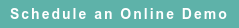# RoyaltyStat Blog

### Posts by Topic

Asset intensity adjustments to operating profits, which I reviewed performing audit assistance, lack economic or statistical merit and are inconsistent with guidance provided in the transfer pricing regulations.

Under US Title 26 CFR 1.482-1(e)(2)(ii): “If material differences exist between the controlled and uncontrolled transactions, adjustments must be made to the results of the uncontrolled transaction if the effect of such differences on price or profits can be ascertained with sufficient accuracy to improve the reliability of the results.” Also, 26 CFR 1.482-1(d)(2): “adjustments must be made to the results of the uncontrolled comparable and must be based on commercial practices, economic principles, or statistical analyses.”

Here, I show how to make asset intensity adjustments to the operating profits of the comparables and the tested party (taxpayer) based on economic and statistical principles.

#### Economic Theory

Let the variables P = OIBAM (operating profits after depreciation but excluding the amortization of acquired intangibles), S = Net Sales, and K = PPENT (property, plant, and equipment (net of depreciation)). The dependent variable OIBAM = OIADP + AM, which is equivalent to operating income [profit] after depreciation and amortization with the addback of the amortization of intangibles.

First, I define the accounting equation:

(1)    S = C + P,

where C = (COGS + XSGA) + DFXA is total cost plus the depreciation of property, plant and equipment.

Cost of goods sold (COGS), Selling, general, and administrative expenses (XSGA, aka Operating expenses), Depreciation of fixed assets (DFXA), and PPENT are Standard & Poor’s Compustat mnemonics. Depreciation in Compustat includes depletion and the amortization of intangibles, DP = DFXA + AM.

RoyaltyStat is a distributor of Compustat Global to which we added interactive (online) transfer pricing analytics, including regression analysis.

The accounting equation (1) is true by definition and is not subject to controversy.

Second, I state a prevalent behavioral equation in which P depends on S and K:

(2)    P = μ S + ρ K + U

where U denotes uncertainty (random error).

The behavioral equation (2) may contain an intercept and may be subject to empirical (factual) controversy, which is resolved by statistical testing using regression analysis.

Third, I combine the two equations (1) and (2) to produce a reduced form single-equation that can be subject to statistical testing based on comparable company financials:

S = C + μ S + ρ K + U
S − μ S = C + ρ K + U
(1 – μ) S = C + ρ K + U

(3)    S = λ1 C + λ2 K + V

where the partial regression coefficients (parameters) are λ1 = 1/(1 – μ) and λ2 = ρ/(1 – μ). The component V is the transformed random variable.

#### Empirical Test

The most reliable method to perform asset intensity adjustments is regression analysis. Otherwise, if regression analysis is not employed, the analyst cannot determine, as required by 26 CFR 1.482-1(e)(2)(ii), if the adjustment “can be ascertained with sufficient accuracy to improve the reliability of the results.”

According to the regression equation (3), an asset adjustment has economic and statistical merit only if (i.e., iff) the regression coefficient λ2 of variable K is different from zero. If the regression coefficient of K is statistically undistinguished from zero, the asset adjustment cannot be made. If λ2 is close to zero (that is, λ2 is not different from zero because its t-statistics is less than 2), then equation (3) is reduced to one-explanatory factor:

(4)     S = λ C + V,

Equation (4) represents the operating profit markup free of an asset intensity adjustment. Equation (3) or (4) is called a Widow's cruse theory of profits from the inexhaustible profits akin to the parable 1 Kings 17: 6-16.

Notice that the sign of the partial regression coefficient λ2 must be determined by an empirical comparability analysis, and not assigned a negative sign by rote.

#### References

Title 26 CFR 1.482

Published on Apr 25, 2022 1:01:10 PM

Ednaldo Silva (Ph.D.) is founder and managing director of RoyaltyStat. He helped draft the US transfer pricing regulations and developed the comparable profits method called TNNM by the OECD. He can be contacted at: esilva@royaltystat.com

RoyaltyStat provides premier online databases of royalty rates extracted from unredacted license agreements
and normalized company financials (income statement, balance sheet, cash flow). We provide high-quality data, built-in analytical tools, customer training and attentive technical support.